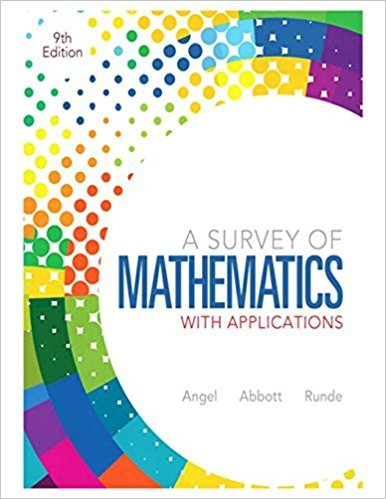×
Log in to StudySoup
Get Full Access to A Survey Of Mathematics With Applications - 9 Edition - Chapter 6.6 - Problem 6.1.313
Join StudySoup for FREE
Get Full Access to A Survey Of Mathematics With Applications - 9 Edition - Chapter 6.6 - Problem 6.1.313

Already have an account? Login here
×
Reset your password

# When both sides of an inequality are multiplied or dividedISBN: 9780321759665 194

## Solution for problem 6.1.313 Chapter 6.6

A Survey of Mathematics with Applications | 9th Edition

• Textbook Solutions
• 2901 Step-by-step solutions solved by professors and subject experts
• Get 24/7 help from StudySoup virtual teaching assistantsA Survey of Mathematics with Applications | 9th Edition

4 5 1 235 Reviews
26
0
Problem 6.1.313

When both sides of an inequality are multiplied or divided by a(n) number, the direction of the inequality symbol is reversed.

Step-by-Step Solution:
Step 1 of 3

T d 1 4- ^so^ Ad ^ s n C3c3 X U3^sinC5Tc\ay oj ^3 . — c doj* 3 do^ k Sin (itU— L- - COS ,* C Y..^ 5o\Adl - 5 c o s 3 x ^ C _ i U) is iKc— " is io\\tr\ . - d u ; =2 dx inside ^unc^ioio^ PW^^WenV^ \^MM •2A" ony. 4Tbe consUn^ noA^bee in+be euab'c z c * t ^ comes ouV andi is inverl-cdn cede Foe d \ cancel tjb

Step 2 of 3

Step 3 of 3

##### ISBN: 9780321759665

A Survey of Mathematics with Applications was written by and is associated to the ISBN: 9780321759665. The answer to “When both sides of an inequality are multiplied or divided by a(n) number, the direction of the inequality symbol is reversed.” is broken down into a number of easy to follow steps, and 21 words. The full step-by-step solution to problem: 6.1.313 from chapter: 6.6 was answered by , our top Math solution expert on 12/28/17, 08:33PM. This full solution covers the following key subjects: . This expansive textbook survival guide covers 105 chapters, and 6968 solutions. This textbook survival guide was created for the textbook: A Survey of Mathematics with Applications, edition: 9. Since the solution to 6.1.313 from 6.6 chapter was answered, more than 268 students have viewed the full step-by-step answer.

Unlock Textbook Solution

Enter your email below to unlock your verified solution to:

When both sides of an inequality are multiplied or divided